Do you like the article?
Share it with others -
Use new possibilities of MetaTrader 5

# Drawing Resistance and Support Levels Using MQL5

25 September 2015, 15:14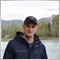5
19 427

### Introduction

First, I will briefly tell you about what support and resistance lines are, how they are built, and how I use them in trading.

All trend figures, lines and models are combinations of support and resistance lines, which underlie the classical trend analysis. Resistance line is based on maximum prices, which occur when traders ("bulls") stop buying currency at a higher price and begin closing open positions for buying. The price of a financial instrument responds to this with a rollback until a similar situation occurs among "bears", i.e. support line is based on minimum prices.

Thus, it can be assumed that maximum points are formed when currency is overbought, and minimum points - when it is oversold. This is the reason why for drawing support and resistance lines I use a standard indicator from the MetaTrader set - Relative Strength Index (RSI), that was developed and published by John Wilder in 1978. This indicator determines overbought and oversold zones of currency.

I use RSI indicator with 8 period; this value is not a result of my observations - this RSI order was recommended by Eric L. Nyman in his book "The Small Trader's Encyclopedia" for all chart periods except for daily and higher. I am completely satisfied with the results of my indicator when operating with RSI (8).

There are two opposing opinions, whether candle wicks should be taken into consideration when searching for maximum and minimum prices (High/Low prices). I, personally, consider them and compare High prices to Low prices when looking for extremum points. If you do not want to take them into consideration, you can simply make minor changes to the indicator code placed below.

Trading with support and resistance lines can occur outside these lines, as well as at price fluctuation inside the figure formed by these lines.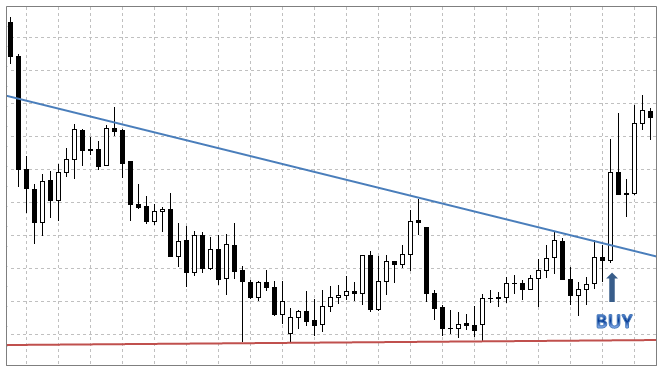I consider the second method to be less effective, especially if price rollback from one of the lines plays a fundamental role in making trading decisions. Figure 1 shows the case, when price, after a rollback from the resistance line, does not reach the support line, turns and breaks through the resistance line. This leads to making a Buy signal stronger. By analyzing angles formed from lines crossing the axis of time, we define a general trend direction, and when the price of financial instrument crosses one of the lines, this allows to make a conclusion about strengthening or reversal of the existing trend. Often, before crossing the support line, for example, the price reaches only up to the middle of the resistance line (this factor enhances a trading signal).

There is a platform called "Autochartist" designed for recognition of trend figures and models. As an experiment, for two months I have been trading with a demo account using signals of price rollback from the resistance or support level - most transactions in this case were closed with loss, unlike trading using signals from breakthrough levels.

Support and resistance levels are also used for determining trend acceleration or deceleration. Increasing the angle of a trend line on a bullish trend implies its acceleration, the appearance of which leads to trend continuation. The angle increase on a bearish trend, on the contrary, indicates trend slowing. A support line (drawn by Low prices) acts as a trend line on a bullish trend, and a resistance line (drawn by High prices) acts as a trend line on a bearish trend.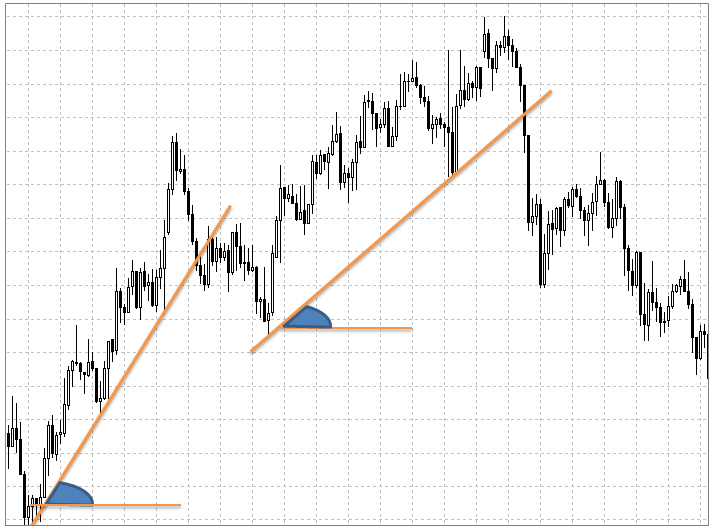Fig.2. Slowing of bullish trend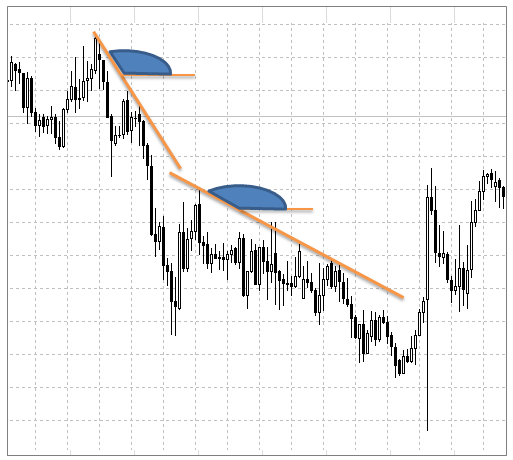Fig.3. Slowing of bearish trend

Trend slowing may suggest its reversal in the nearest future.

Analysis of chart figures, formed by support and resistance lines, is one of the oldest methods of technical analysis. From my trading experience on the Foreign Exchange market, I can state that the above mentioned method is not only old, but is also effective. I believe that a lot of traders use it. Building support and resistance lines manually with MetaTrader 5 terminal is an easy task for any trader who has theoretical knowledge. But it is not the case when creating a program that draws these lines automatically.

In this article, I want to propose my own method of drawing support and resistance lines which can be implemented with both MQL5 and MQL4. Hopefully, you will find this information useful.

### Earlier presented methods of drawing support and resistance levels in brief

Most of the time, quotes for currency pairs range within resistance and support lines — this fact underlies the article of Gleb Slobodov on how to draw these lines. As a result, we have two horizontal levels that define a range of price fluctuations. A breakthrough of one of them, probably, gives us a signal to buy or sell. However, the proposed principle has several disadvantages:

• The number of analyzed bars is selected manually and this parameter sets a price range, hence value of resistance and support levels.
• The process of drawing levels is not fully automated and therefore eliminates the possibility of creating an automated trading system.
• Using this method, we obtain horizontal levels that don't allow us to analyze trend's direction and strength.

The indicator of support and resistance levels used in the trading strategy of Igor Gerasko also defines horizontal levels.

In this article, I want to introduce to you to the principle of drawing support and resistance lines, allowing to determine trend direction, formation of trend models and figures. The lines are drawn based on minimum and maximum extremum points.

### Principle of finding extremums

Determining maximum and minimum prices at a given period of time does not pose any difficulties. It is important to choose length of analyzed chart (time interval) right, that it is constantly changing and therefore can not be set manually. To find this currency chart section, I'll use Relative Strength Index (RSI) indicator, that is included in the standard set of indicators of MetaTrader 5 terminal.

Overbought and oversold levels are determined according to levels of RSI indicator. At these moments the chart of our currency pair deviates from its direction (trend) leading to a price rollback. Specifically here our extremums will be formed, at these gaps we will be searching for minimum and maximum prices.

As an oversold level, I will take the RSI indicator value equal to 35; overbought level - 65 (upper and lower levels are equally positioned from the middle of RSI = 50). The period of RSI indicator equals 8.

It should be noted, that often during a strong trend, for example, increasing trend, RSI repeatedly crosses the upper level, but fails to reach the bottom. This leads to a necessity of level adjustment, which does not correspond to the trend direction.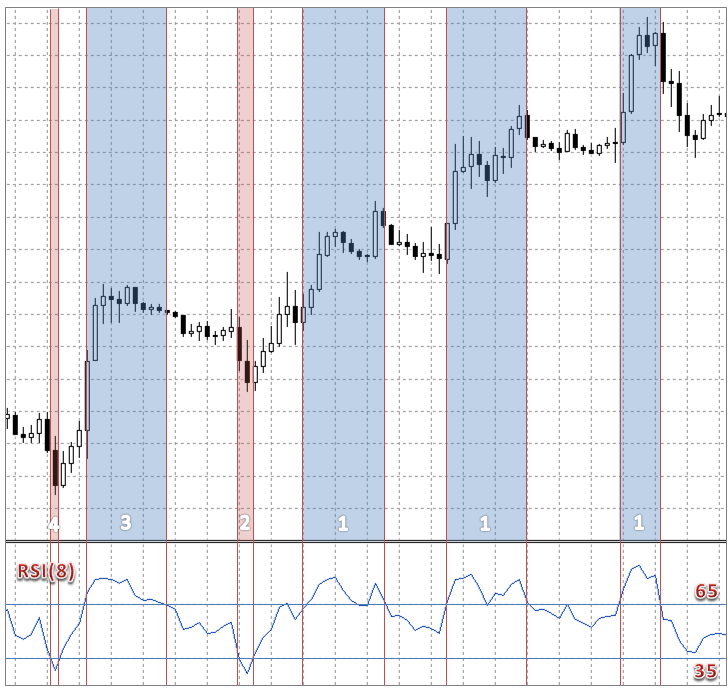Fig.4. Search zones for extremum bars

In the figure above, I have designated a search zone for extremum bars: first, second, third and fourth, respectively, with numbers 1, 2, 3 and 4. Before crossing a low boundary (RSI = 35), the RSI indicator, starting from the current bar, is included in the overbought zone (RSI >= 65) three times, therefore there will be three time intervals for searching for the first extremum. Further, search zones are in order being determined for consequent three bars.

In zones 1 and 3, I will look for bars with highest prices and in zones 2 and 4 for lowest prices. As a result, we will obtain 4 extremum points — by combining them with rays, we will get the ascending price channel.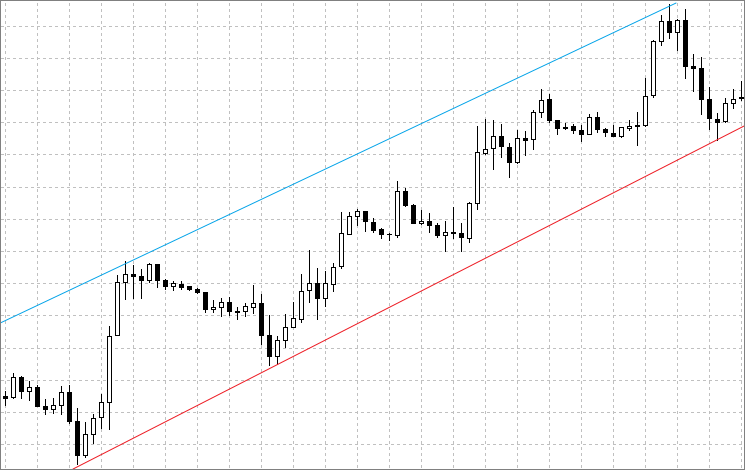Fig.5. Ascending channel

If you paid attention, you probably have noticed that there were only few bars for searching minimum prices, only 4 (2 for each zone). Due to the predominant uptrend, the RSI indicator has barely touched the minimum level, while outside RSI = 65 level the indicator was almost the same time as inside the channel 35 < RSI < 65. Therefore, the indicator code that I have presented in this article for finding points №2 and 4, will use the RSI level shifted towards the middle (towards 50). Depending on what was the first extremum (minimum or maximum), high or low RSI level will be set.

All currency pairs, all periods of RSI level chart value and value of one's subsequent deviation, are selected experimentally. I consider this to be a disadvantage of my system for determining extremums.

### Function for finding extremum bar with the lowest index (first bar) Ext_1

The function for finding extremum bars, which I called "Ext_1", has the following input parameters:

```int Ext_1(double low,      //low RSI level, oversold level
double high,     //high RSI level, overbought level
int bars,        //number of analyzed bars, to avoid copying unnecessary data
//possible to set bars = 300
int h_rsi,       //handle of RSI indicator
string symbol,   //symbol of chart
float distans,   //distance for deviation of one of indicator levels
//allows to define search boundaries of first extremum bar
```

The first two function's input parameters are the parameters of the RSI indicator. They play no role in calculation of the indicator line and are used only for convenience of visual evaluation value (indicator line's location in regards to given levels). I use these levels to determine price ranges, among which I will search for minimum and maximum values. Parameters "low" and "high" ​​obtain values of relevant external variables set in the indicator code on the global level:

```input double Low_RSI = 35.0; // Low RSI level for finding extremum
input double High_RSI= 65.0; // High RSI level for finding extremum
```

The input parameter "bars" sets the number of elements copied into arrays containing Low and High bar prices as well as values ​​of the RSI indicator:

```double m_rsi[],m_high[],m_low[];                              //initialization of arrays
int h_high = CopyHigh(symbol, period_trade, 0, bars, m_high); //fill array of high candle prices
int h_low = CopyLow(symbol, period_trade, 0, bars, m_low);    //fill array of low candle prices
if(CopyBuffer(h_rsi, 0, 0, bars, m_rsi)<bars)                 //fill array with indicator RSI data
{
Print("Failed to copy indicator buffer!");
}
```

Arrays m_rsi[],m_high[] and m_low[] have reverse indexing order:

```ArraySetAsSeries(m_rsi,true);
ArraySetAsSeries(m_high,true);
ArraySetAsSeries(m_low,true);
```

As I said previously, with prevalence of a bullish trend, the line of RSI indicator, which has a range of values ​​from 0 to 100, most of the times will have a value of > 50. At times when minimum point is formed, the RSI value will be less remote from the center (50), than during the formation of the maximum point. Therefore, at the first intersection of one of the indicator values (low or high), another value has to be shifted closer to the middle. The magnitude of deviation is determined by the input parameter "distans", which is given the value of an external variable:

`input float Distans=13.0;    // Deviation of RSI level`

The input parameter "h_rsi" of "Ext_1" function gets the value of RSI handle obtained during indicator initialization with function iRSI():

`h_RSI=iRSI(Trade_Symbol,Period_Trade,Period_RSI,PRICE_CLOSE);  //return handle of RSI indicator`

Variables Trade_Symbol and Period_Trade are initialized on the global level and contain information about the currency pair and chart period, accordingly. The variable Period_RSI contains the value of the RSI indicator period specified in the external parameters of my indicator:

`input uchar Period_RSI =8;   // RSI period`

Once we have created and filled arrays containing High and Low bar prices, as well as value of the RSI indicator, corresponding to these bars, you can proceed to the actual search of the first extremum. In order to determine where will the first extremums be (support or resistance line) and to stop the bar analysis at the right moment, two variables of type bool are required:

```bool ext_max = true;    //bool type variables are used to stop
bool ext_min = true;    //bar analysis at the right time
```

Value ext_max = true permits the search of maximum extremum, value ext_min = true, respectively, permits the search of minimum extremum. At the first intersection of RSI indicator with one of the levels (low or high), value of one of bool variables is changed to false, and the intersection of RSI level, whose bar analysis is disabled, means that the required number of bars is analyzed, and the first extremum is found.

If, when analyzing the first bar, the value of the RSI indicator is outside one of its levels, it is likely that the formation of the price range in which extremum has to be searched for, is not yet complete, and there is no point in analyzing it. The analysis of such bars should be excluded. On the figure below I have highlighted the price range when the analysis is not conducted (take note of the position of the RSI indicator line relative to the upper level):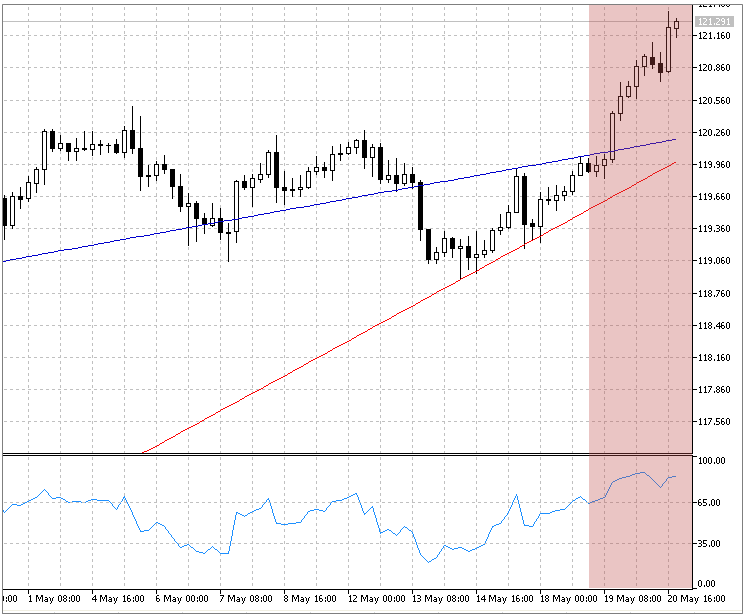Fig.6. Disable analysis at the incomplete price range

To implement such operation, it is required to create another variable of type bool:

`bool flag=false;`

To determine maximum and minimum prices among the analyzed bars, it is required to create the following additional variables of type double:

```double min = 100000.0;  //variables to identify maximum and minimum prices
double max = 0.0;       //...
```

The whole loop for bar search with first extremum will be the following:

```for(int i=0;i<bars;i++) //bar loop
{
double rsi = m_rsi[i];                                   //get RSI indicator value
double price_max = NormalizeDouble(m_high[i], digits);   //High prices
double price_min = NormalizeDouble(m_low[i], digits);    //Low prices of selected bar
if(flag==false) //condition to avoid searching for extremum on incomplete trend
{
if(rsi<=low||rsi>=high) //if first bars in overbought zones or oversold zones,
continue;            //then move to next bar
else flag = true;       //if not, proceed with analysis
}
if(rsi<low) //if found crossing of RSI with low level
{
if(ext_min==true) //if RSI hasn't crossed high level
{
if(ext_max==true) //if searching for maximum extremum hasn't been disabled
{
ext_max=false;     //then disable searching for maximum extremum
if(distans>=0) high=high-distans; //change high level, on which then
}                                    //second bar search will be executed
if(price_min<min) //search and memorise first bar index
{                 //comparing Low candle prices
min=price_min;
index_bar=i;
}
}
else break; /*Exit loop since searching for minimum extremum is already disabled,
it means the maximum is found*/
}
if(rsi>high) //further, algorithm is the same, only in search for maximum extremum
{
if(ext_max==true)
{
if(ext_min==true)
{
ext_min=false;
if(distans>=0) low=low+distans;
}
if(price_max>max)
{
max=price_max;
index_bar=i;
}
}
else break; /*Exit loop since searching for maximum extremum is disabled,
it means the minimum is found*/
}
}
```

### General function for finding all subsequent extremums Ext_2

This function will receive the same input parameters as the Ext_1 function, plus three other important parameters:

1. The parameter that contains a reference to a structure where indexes of extremum bars will be saved. It is required to determine from which index bar the search of extremum will begin.
2. The serial number of the extremum bar that has to be found (takes value from 2 to 4). It is required to choose the index of the desired bar from the structure, as well as to determine, on which line (support or resistance) the desired extremum will be located.
3. The bool type parameter that determines on which line (support or resistance) the first extremum bar is located. Without this information, it is impossible, based on the serial number, to determine on which line the desired bar should be located.
```int Ext_2(double low,    //low RSI level, oversold level
double high,   //high RSI level, overbought level
int bars,      //number of analyzed bars, to avoid copying unnecessary data into arrays
int h_rsi,     //handle of RSI indicator
string symbol, //symbol of chart
st_Bars &bars_ext,//structure containing codes of found bars
char n_bar,    //ordinal number of bar required to find (2,3 or 4)
float distans, //distance for deviation of one of indicator levels
bool first_ext,//type of first bar
```

On the global level we create a structure type that will contain indexes of all 4 extremum bars:

```struct st_Bars //structure initialization
{
int               Bar_1;
int               Bar_2;
int               Bar_3;
int               Bar_4;
};

st_Bars Bars_Ext; //declaration of structure type variable
```

To determine the indicator line where the desired extremum will be located, first, two variables of type bool have to be created:

```bool high_level= false; //variables to determine type of desired bar
bool low_level = false; //...
```

If the ordinal number of the desired extremum bar equals 2 or 4, and the first extremum bar lies on the support line, the desired bar must lie on the resistance line and, therefore, it is required to analyze bars, whose value of RSI is higher or equals the upper level (high parameter). If the desired extremum bar's ordinal number equals 3, and the first extremum bar is located on the support line, then the desired bar will also be located on this line. If the first extremum bar is on the resistance line, then the position of the desired bar is chosen accordingly.

```if(n_bar!=3)
{
if(first_ext==true)//if first point was maximum
{
low_level=true;//then this should be minimum
if(distans>=0) low=low+distans; //if necessary, displace low level of RSI
}
else //if minimum
{
high_level = true;
if(distans>=0) high=high-distans; //if necessary, displace high level of RSI
}
}
else
{
if(first_ext==false)//if first point was minimum
{
low_level=true;//then this point has to be minimum
if(distans>=0) high=high-distans; //if necessary, displace high level of RSI
}
else //if maximum
{
high_level = true;
if(distans>=0) low=low+distans; //if necessary, displace low level of RSI
}
}
```

Another variable of type bool is needed to determine the moment when the desired extremum is found and the bar analysis can be stopped.

`bool _start = false;    `

The value of this variable is changed to true, when we find the required bar range for analysis in history. Bar analysis is terminated, if _start = true, and when low_level = true, the RSI indicator line crosses the high level, and when high_level = true, the RSI indicator line crosses the low level.

```if(_start==true && ((low_level==true && rsi>=high) || (high_level==true && rsi<=low)))
break; //exit loop if second extremum is found, and RSI crossed opposite level
```

The loop for extremum bar search will be the following:

```for(int i=bar_1;i<bars;i++) //analyze remaining bars
{
rsi=m_rsi[i];
price_max = NormalizeDouble(m_high[i], digits);
price_min = NormalizeDouble(m_low[i], digits);
if(_start==true && ((low_level==true && rsi>=high) || (high_level==true && rsi<=low)))
{
break; //exit loop if second extremum is found, and RSI crossed opposite level
}
if(low_level==true) //if looking for minimum extremum
{
if(rsi<=low)
{
if(_start==false) _start=true;
if(price_min<min)
{
min=price_min;
index_bar=i;
}
}
}
else //if looking for maximum extremum
{
if(rsi>=high)
{
if(_start==false) _start=true;
if(price_max>=max)
{
max=price_max;
index_bar=i;
}
}
}
}
```

The variable Bar_1 contains the index of the previous extremum bar, which is calculated using the switch operator:

```switch(n_bar) //find index of previous bar
{
case 2: bar_1 = bars_ext.Bar_1; break;
case 3: bar_1 = bars_ext.Bar_2; break;
case 4: bar_1 = bars_ext.Bar_3; break;
}
```

To find out on what indicator line (support or resistance) the first extremum bar is located, it is sufficient to get its index and the RSI indicator value on the bar with obtained index:

```bool One_ext (st_Bars & bars_ext, // variable of structure type to obtain first bar index
string symbol,     //symbol of chart
int h_rsi,         //handle of indicator
double low,        //set oversold level of RSI (high level can be used)
{
double m_rsi[];               //array initialization of indicator data
ArraySetAsSeries(m_rsi,true); //indexing
CopyBuffer(h_rsi,0,0,bars_ext.Bar_1+1,m_rsi); //fill array with RSI data
double rsi=m_rsi[bars_ext.Bar_1]; //define RSI value on bar with first extremum
if(rsi<=low)                      //if value is below low level,
return(false);                 //then first extremum is minimum
else                              //if not,
return(true);                     //then maximum
}
```

### Processing the results

Now we know the indexes of all four bars and their corresponding prices (low or high). In order to fill the arrays that will match the value of the indicator lines on each bar, it is required to get the equations of two lines corresponding to resistance and support lines. The well-known line equation used for this purpose is: y = kx + b. In our case, "x" is a bar index, and "y" is a price (for support line - low candle price, for resistance line - high candle price).

To find values ​​of the "k" and "b" coefficients it is sufficient to substitute the corresponding values ​​of two known extremum bars in the line equation, and to combine the obtained expressions in the equation system. As a result, we obtain the following expressions on the system:

```K=(price_2-price_1)/(_bar2-_bar1);  //find coefficient K
B=price_1-K*_bar1;                  //find coefficient B
```

where

`double K,B;`

"K" and "B" are global variables which correspond to values ​​of the coefficients "k" and "b" in the line equation;

`int _bar1,_bar2;`

These are bar indexes located on the same line;

`double price_1,price_2;`

these are low prices of the respective bars, if required to define "K" and "B" support lines or high price of the respective bars, you need to determine "K" and "B" for the resistance line.

The function presented below sets values ​​of global variables "K" and "B" for the support line, if the parameter "_line" is false, and for the line of resistance, if the parameter "_line" is true:

```void Level(bool _line,              //parameter that defines resistance/support line, which coefficients have to be found
bool _first_ext,         //type of first extremum (already familiar to you)
st_Bars &bars_ext,       //structure that contains bar indexes
string _symbol,          //symbol
ENUM_TIMEFRAMES _period) //period of chart
{
int bars=Bars_H;           //number of analyzed bars
double m_high[],m_low[];         //initialization of arrays
ArraySetAsSeries(m_high,true);   //arrays are indexed from first element
ArraySetAsSeries(m_low,true);    //...
int h_high = CopyHigh(_symbol, _period, 0, bars, m_high); //fill array of High candle price
int h_low = CopyLow(_symbol, _period, 0, bars, m_low);    //fill array of Low candle price
double price_1,price_2;
int _bar1,_bar2;
int digits=(int)SymbolInfoInteger(_symbol,SYMBOL_DIGITS);//number of decimal places in current symbol
if(_line==true)                                          //if resistance line is required
{
if(_first_ext==true) //if first extremum is maximum
{
price_1 = NormalizeDouble(m_high[bars_ext.Bar_1], digits);
price_2 = NormalizeDouble(m_high[bars_ext.Bar_3], digits);
_bar1 = bars_ext.Bar_1;
_bar2 = bars_ext.Bar_3;
}
else                                                  //if minimum
{
price_1 = NormalizeDouble(m_high[bars_ext.Bar_2], digits);
price_2 = NormalizeDouble(m_high[bars_ext.Bar_4], digits);
_bar1 = bars_ext.Bar_2;
_bar2 = bars_ext.Bar_4;
}
}
else                                                     //if support line is required
{
if(_first_ext==true) //if first extremum is maximum
{
price_1 = NormalizeDouble(m_low[bars_ext.Bar_2], digits);
price_2 = NormalizeDouble(m_low[bars_ext.Bar_4], digits);
_bar1 = bars_ext.Bar_2;
_bar2 = bars_ext.Bar_4;
}
else                                                  //if minimum
{
price_1 = NormalizeDouble(m_low[bars_ext.Bar_1], digits);
price_2 = NormalizeDouble(m_low[bars_ext.Bar_3], digits);
_bar1 = bars_ext.Bar_1;
_bar2 = bars_ext.Bar_3;
}
}
K=(price_2-price_1)/(_bar2-_bar1);  //find coefficient K
B=price_1-K*_bar1;                  //find coefficient B
}
```

The line equation is: y = kx + b, where financial instrument price is used for axis "y", and bar index for axis ​"x". If for axis "x" we use the number of seconds passed since 1st of January 1970, the line chart in the day-off area will show "chaotic" results, which is the reason I used the index bars.

From the "OnCalculate" function, the "Level" function is called twice: first time before filling the array for the resistance line, and second time to fill the array with price values ​​for the support line:

```for(int i=0;i<Bars_H;i++)
{
resistanceBuffer[i]=NormalizeDouble(K*i+B,Dig);
}
for(int i=0;i<Bars_H;i++)
{
supportBuffer[i]=NormalizeDouble(K*i+B,Dig);
}
```

### Example of an indicator displaying support and resistance levels

The result of the indicator operation that uses all of the above functions and draws the support and resistance lines is the following: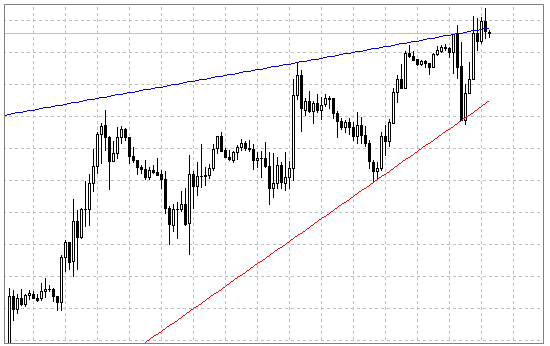Fig.7. The result of indicator operation

The indicator is constructed in a such way that after the formation of a new extremum bar, array values ​​of support and resistance lines can be changed, and then levels will be automatically redrawn. However, if we calculate and memorize the angle of one of the lines to the time axis on the chart, and then compare it with the new angle of the same line, it is possible to conclude of a trend acceleration and deceleration, as already mentioned in this article.

The full code of the indicator is in the file attached to this article.

### Conclusion

Certainly, it is much easier to build these lines manually, as you don't need to select the indicator parameters for each symbol and period. However, this indicator can serve as a basis or a part of the strategy laid down in the automatic trading system. After receiving a data array of indicator lines, you can analyze the angle of inclination, direction of the trend, as well as identify the graphic shape formed by these lines. All this, ultimately, makes it possible to analyze Buy or Sell signal strength, trade inside the price channel as well as on the breakthrough of support and resistance lines.

Translated from Russian by MetaQuotes Software Corp.
Original article: https://www.mql5.com/ru/articles/1742

Attached files |
s_rind.mq5 (34.78 KB)
Last comments | Go to discussion (5)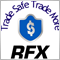| 25 Sep 2015 at 17:55
A very good idea for automating the process of identifying support and resistance levels. What is the best RSI period which is suitable for using this method on Daily charts?| 27 Sep 2015 at 13:32
A very good idea for automating the process of identifying support and resistance levels. What is the best RSI period which is suitable for using this method on Daily charts?
Hello! I use RSI period equal 8. I often use parameter " Deviation of RSI level" on D1= 15,0.| 4 Oct 2015 at 21:14
Nice article.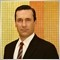| 12 Nov 2015 at 20:05
Very good article, God bless you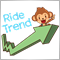| 12 Nov 2017 at 11:26

Article very interesting. Thanks for your work and for sharing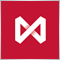How to Secure Your Expert Advisor While Trading on the Moscow Exchange
The article delves into the trading methods ensuring the security of trading operations at the stock and low-liquidity markets through the example of Moscow Exchange's Derivatives Market. It brings practical approach to the trading theory described in the article "Principles of Exchange Pricing through the Example of Moscow Exchange's Derivatives Market".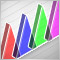An Introduction to Fuzzy Logic
Fuzzy logic expands our boundaries of mathematical logic and set theory. This article reveals the basic principles of fuzzy logic as well as describes two fuzzy inference systems using Mamdani-type and Sugeno-type models. The examples provided will describe implementation of fuzzy models based on these two systems using the FuzzyNet library for MQL5.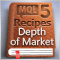MQL5 Cookbook: Implementing Your Own Depth of Market
This article demonstrates how to utilize Depth of Market (DOM) programmatically and describes the operation principle of CMarketBook class, that can expand the Standard Library of MQL5 classes and offer convenient methods of using DOM.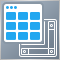Using Layouts and Containers for GUI Controls: The CGrid Class
This article presents an alternative method of GUI creation based on layouts and containers, using one layout manager — the CGrid class. The CGrid class is an auxiliary control that acts as a container for other containers and controls using a grid layout.